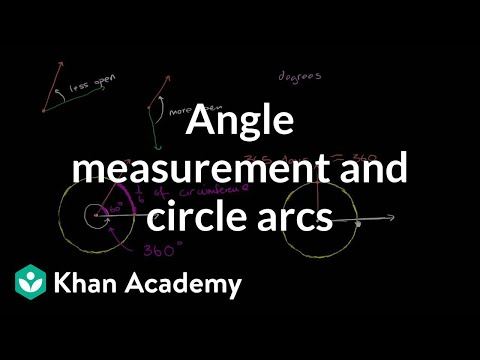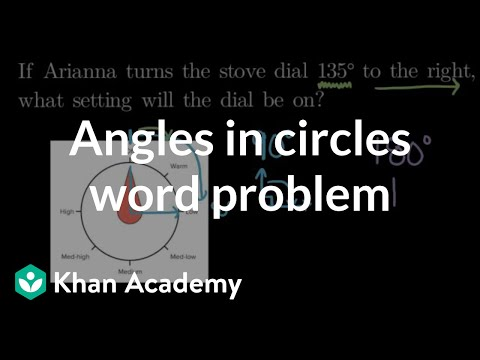Video

# Identifying an angle (Full video)

Description: Sal matches pictures of angles to their degrees. Created by Sal Khan. This angle right over here, it makes a complete line. So it makes sense that this is 30, this is 60, and it also makes sense that this angle is 1/3 the measure of this angle right over here, that this is 90 degrees. And then if you were to double this, then this part of the angle would go all the way out, and you would get to the 180 degrees.

### Other videos you might be interested in### Angle measurement & circle arcs (Full video)